Home
Hostname: page-component-5c569c448b-s84wp Total loading time: 0.218 Render date: 2022-07-04T22:01:22.862Z Has data issue: true Feature Flags: { "shouldUseShareProductTool": true, "shouldUseHypothesis": true, "isUnsiloEnabled": true, "useRatesEcommerce": false, "useNewApi": true } hasContentIssue true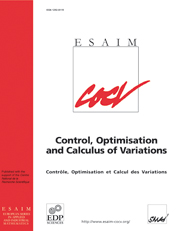ESAIM: Control, Optimisation and Calculus of Variations

# The geometrical quantity in damped wave equations on a square

Published online by Cambridge University Press:  11 October 2006

## Abstract

The energy in a square membrane Ω subject to constant viscous damping on a subset $\omega\subset \Omega$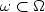decays exponentially in time as soon as ω satisfies a geometrical condition known as the “Bardos-Lebeau-Rauch” condition. The rate $\tau(\omega)$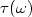of this decay satisfies $\tau(\omega)= 2 \min( -\mu(\omega), g(\omega))$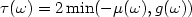(see Lebeau [Math. Phys. Stud.19 (1996) 73–109]). Here $\mu(\omega)$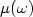denotes the spectral abscissa of the damped wave equation operator and $g(\omega)$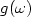is a number called the geometrical quantity of ω and defined as follows. A ray in Ω is the trajectory generated by the free motion of a mass-point in Ω subject to elastic reflections on the boundary. These reflections obey the law of geometrical optics. The geometrical quantity $g(\omega)$is then defined as the upper limit (large time asymptotics) of the average trajectory length. We give here an algorithm to compute explicitly $g(\omega)$when ω is a finite union of squares.

Type
Research Article
Information

## Access options

Get access to the full version of this content by using one of the access options below. (Log in options will check for institutional or personal access. Content may require purchase if you do not have access.)

## References

Asch, M. and Lebeau, G., The spectrum of the damped wave operator for a bounded domain in $\mathbb{R}^2$. Experiment. Math. 12 (2003) 227241. CrossRef
Bardos, C., Lebeau, G. and Rauch, J., Sharp sufficient conditions for the observation, control and stabilization of waves from the boundary. SIAM J. Control Optim. 30 (1992) 10241065. CrossRef
Benaddi, A. and Rao, B., Energy decay rate of wave equations with infinite damping. J. Diff. Equ. 161 (2000) 337357. CrossRef
Carlos, C. and Cox, S., Achieving arbitrarily large decay in the damped wave equation. SIAM J. Control Optim. 39 (2001) 17481755.
A. Chambert-Loir, S. Fermigier and V. Maillot, Exercices de mathématiques pour l'agrégation. Analyse I. Masson (1995).
Cox, S. and Zuazua, E., The rate at which energy decays in a damping string. Comm. Partial Diff. Equ. 19 (1994) 213243. CrossRef
P. Hébrard, Étude de la géométrie optimale des zones de contrôle dans des problèmes de stabilisation. Ph.D. Thesis, University of Nancy 1 (2002).
Hébrard, P. and Henrot, A., Optimal shape and position of the actuators for the stabilization of a string. Syst. Control Lett. 48 (2003) 119209. CrossRef
G. Lebeau, Équation des ondes amorties, in Algebraic and Geometric Methods in Mathematical Physics. Kluwer Acad. Publ., Math. Phys. Stud. 19 (1996) 73–109.
Rauch, J. and Taylor, M., Decay of solutions to nondissipative hyperbolic systems on compact manifolds. Comm. Pure Appl. Math. 28 (1975) 501523. CrossRef
Sjostrand, J., Asymptotic distribution of eigenfrequencies for damped wave equations. Publ. Res. Inst. Sci. 36 (2000) 573611. CrossRef
S. Tabachnikov, Billiards mathématiques. SMF collection Panoramas et synthèses (1995).
Zuazua, E., Exponential decay for the semilinear wave equation with localized damping. Comm. Partial Equ. 15 (1990) 205235.

# Save article to Kindle

Note you can select to save to either the @free.kindle.com or @kindle.com variations. ‘@free.kindle.com’ emails are free but can only be saved to your device when it is connected to wi-fi. ‘@kindle.com’ emails can be delivered even when you are not connected to wi-fi, but note that service fees apply.

Find out more about the Kindle Personal Document Service.

The geometrical quantity in damped wave equations on a square
Available formats
×

# Save article to Dropbox

To save this article to your Dropbox account, please select one or more formats and confirm that you agree to abide by our usage policies. If this is the first time you used this feature, you will be asked to authorise Cambridge Core to connect with your Dropbox account. Find out more about saving content to Dropbox.

The geometrical quantity in damped wave equations on a square
Available formats
×

# Save article to Google Drive

To save this article to your Google Drive account, please select one or more formats and confirm that you agree to abide by our usage policies. If this is the first time you used this feature, you will be asked to authorise Cambridge Core to connect with your Google Drive account. Find out more about saving content to Google Drive.

The geometrical quantity in damped wave equations on a square
Available formats
×
×